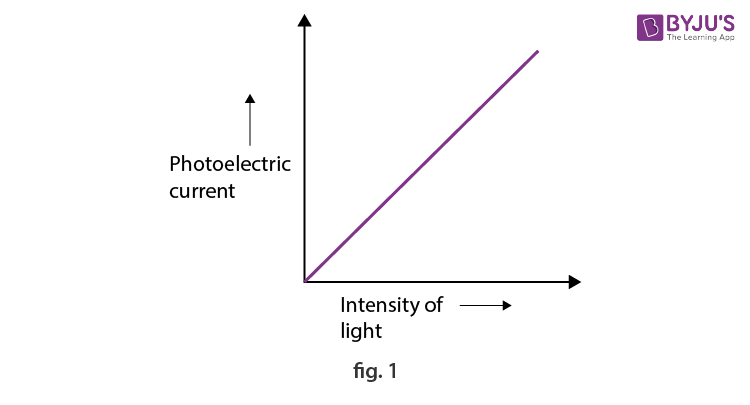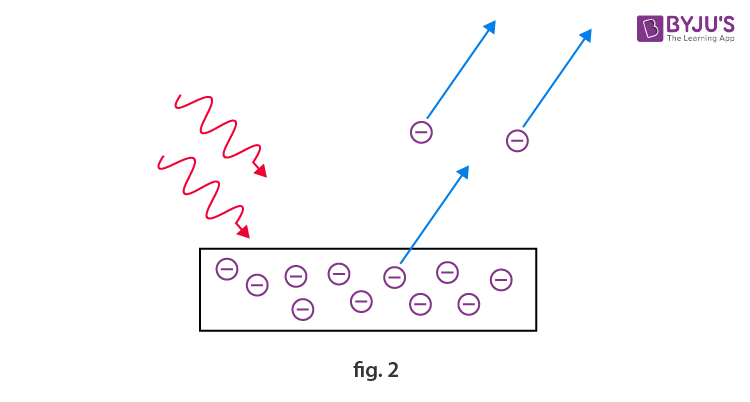# Dual Nature of Matter

Dual nature of matter is an important concept in JEE physics and is basically the study of different nature that a matter possesses or exhibits. A matter can either display or have a particle nature or wave nature. Various experiments have further been conducted to prove this theory.

Initially, the properties of matter or light were explained in terms of its particle nature. Corpuscular theory of light, etc. were some of the primitive steps that influenced this. Later on, it was experimentally found out that matter does possess the properties of a wave. Hence, the matter is said to possess dual nature, i.e., it has both the properties of a particle and as well as a wave.

Maxwell’s equation of electromagnetism and Hertz experiments on the generation and detection of electromagnetic waves in 1887 strongly established the wave nature of light.

Thus, the wave-particle duality is an important concept in quantum mechanics which describes that every particle or more specifically quantum entity may be expressed as either a particle or a wave. This concept further helps to modify the inability of the classical mechanic’s approach or theories to fully describe the behaviour of the matter.

## Important Topics Covering Dual Nature of Matter

Here are some of the important topics that should be learnt while dealing with this concept.

Electronic Emission

The minimum energy that is required to emit an electron from the surface of a metal can be supplied to the free electrons by either of the methods given below:

• Thermionic Emission: Required thermal energy is provided to the free electrons by suitably heating it so as to enable them to come out of the metal.
• Field Emission: Electrons are kept under the strong influence of the electric field to emit the electron out of the metal.
• Photo-electric Emission: When the light of appropriate frequency is made to illuminate a metal surface, electrons are emitted from it. These photo-generated electrons are called Photoelectrons.

Photoelectric Effect

The photoelectric effect is a phenomenon that involves electrons escaping from the surface of materials. Usually, the surface of the material is composed of both positive and negative ions. What happens in the process is when light is made to be incident on the metal surface, some of the electrons which are present near the surface will absorb enough energy from the incident radiation and thus overcomes the attraction of the positive ions. Further, when the electrons gain as much sufficient energy required, they will escape out of the metal surface into the surrounding space. This forms the basis for the Photoelectric effect.

Some related terms include:

• Work Function: The minimum energy that is required to eject an electron from a metal surface.
• Threshold Frequency: The minimum frequency of light that can force an electron to emit from a metal surface.
• Threshold Wavelength: The maximum wavelength of light that can eject a photoelectron from the surface of a metal.

If, Work Function is denoted by Ɵ, Threshold Frequency by f, and Threshold Wavelength by ƛ; then we have Ɵ = hf =hc/ ƛ; where h is Planck’s Constant. E=hf

Concepts to understand:

Cut-off Potential: The minimum negative or retarding potential V0 given to a plate for which photoelectric current becomes zero is called Cut-off or Stopping Potential.

Effect of Intensity of incident light is linear with the photoelectric current for a fixed incident frequency as depicted in Figure 1 given below.

Effect of Potential on Photoelectric Current is that it increases with increase in Potential applied to the collector for a fixed frequency and intensity of incident light, and finally attains maximum current termed as Saturation Current.

### Laws Of Photoelectric Effect

The laws of the Photoelectric effect are as follows:

1. For a given metal and frequency of incident light, the photoelectric current is directly proportional to the intensity of incident light.

2. For a given metal, there is a certain minimum frequency, called Threshold frequency, below which there is no photo-electric emission.

3. The maximum kinetic energy of photoelectrons above a threshold frequency depends upon the frequency of incident light.

4. The photoelectric emission is an instantaneous process.The minimum negative potential given to anode plate at which photoelectric current becomes zero is called Stopping Potential (V0).Figure 1 shows the plot between Photoelectric Current and Intensity of light. Figure 2, on the other hand, represents the diagram for Photoelectric effect where the ejection of electrons (marked in blue) from the metal surface is depicted when the light of suitable intensity (marked with red) is incident over it.

To find out the relation between Stopping Potential (V0) and Threshold Frequency f, we have:

hf= KE + W (KE is maximum Kinetic Energy=eV0 and W is the work function=hf0).

KE= hf – W =hf – hf0 = h (f- f0)

Or, eV0 = h (f- f0)

V0 = (h/e) (f- f0) = (h/e) [(c/ƛ) – (c/ƛ0)] = (hc/e) [(1/ƛ) – (1/ƛ0)].

For photoelectric emission, ƛ < ƛ0 and f > f0.

## De Broglie Hypothesis

With respect to Quantum theory of matter, De Broglie postulated the relationship between momentum and wavelength. Mathematically, it is given in the form, wavelength ƛ = h/P; where P is the momentum of the particle under study and h is Planck’s Constant.

### De Broglie-Bohm Theory

De Broglie-Bohm Theory, which is also called as Bohmian Mechanics considers the wave nature predominates and thus the particle-wave duality goes vanished. It explains wave behaviour as a scattering with wave appearance as the particle’s expression is subjected over to a guiding equation or quantum potential.

### Derivation Of De Broglie Equation

hf=mc2

We know that the frequency f=c/ ƛ

It implies that hc/ ƛ=mc2 or ƛ = h/mc

If c=v; then ƛ=h/mv

We also know the momentum of a particle, P= mv.

Hence, ƛ=h/P.

## Heisenberg’s Uncertainty Principle

Heisenberg’s Uncertainty Principle states that both the momentum and position of a particle cannot be determined simultaneously.

Mathematically, it can be expressed as ∆ x ∆P ≥ (h / 4π) where ∆x represents the Uncertainty in position, ∆P represents the Uncertainty in Momentum.

## Planck’s Quantum Theory

When we apply heat to a black body, it results in the emission of thermal radiations having different wavelengths or frequency. As such Max Planck put forward a theory called Planck’s Quantum Theory to explain these radiations. The main highlights of the theory include:

• Substances absorb or radiate energy in a discontinuous manner. This process takes place in the form of small packets.
• The above-said process takes place in whole-number multiples of quantum as hf, 2hf, 3hf……nhfs; where n is a positive integer.
• The smallest packet of energy is called Quantum. It is referred to as Photon in case of Light.
• The energy of a Quantum is directly proportional to the radiation frequency.

## Electron Under An Electric Field

Consider an electron of mass m, with a charge q being accelerated through a potential V from rest. The kinetic energy K of the electron equals the work done on it by the electric field which equals to qV.

K = qV = ½ mv2 = P2/2m

P = √ 2mK = √ 2mqV

The de Broglie wavelength ƛ of the electron is given by;

ƛ = h / P = h / √2mK = h / √2mqV

Substituting the numerical values of h, m and e; we have ƛ=1.227/ nm; where V is the magnitude of accelerating potential in Volts.

## Davisson And Germer Experiment

Davisson and Germer Experiment, for the first time, proved the wave nature of electrons and verified the de Broglie equation. The results established the first experimental proof of quantum mechanics.

The experimental setup for Davisson and Germer Experiment is enclosed within a vacuum chamber. Thus, the deflections and scattering of electrons by the medium are prevented. The main parts consist as given below:

• Electron Gun: It is a Tungsten filament that emits electrons via thermionic emission.
• Electrostatic Particle Accelerator: Two oppositely charged plates are employed to accelerate the electrons at a known potential.
• Collimator: The accelerator is enclosed within a cylinder that has a narrow beam for the electrons along its axis.
• Target: It is a Nickel crystal over which the electron beam is fired.
• Detector: It is used to capture the scattered electrons from the Ni Crystal.
Test Your Knowledge on Dual Nature Of Matter